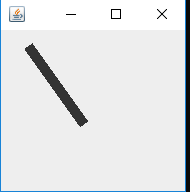# Java Applet | Draw a line using drawLine() method

• Difficulty Level : Basic
• Last Updated : 18 Jan, 2019

This article shall be explaining the code to draw a line using paint in Java. This uses drawLine() method.

Syntax:

`drawLine(int x1, int y1, int x2, int y2)`

Parameters: The drawLine method takes four arguments:

• x1 – It takes the first point’s x coordinate.
• y1 – It takes first point’s y coordinate.
• x2 – It takes second point’s x coordinate.
• y2 – It takes second point’s y coordinate

Result: This method will draw a line starting from (x1, y1) co-ordinates to (x2, y2) co-ordinates.

Below programs illustrate the above problem:

Example:

 `// Java program to draw a line in Applet ` ` `  `import` `java.awt.*; ` `import` `javax.swing.*; ` `import` `java.awt.geom.Line2D; ` ` `  `class` `MyCanvas ``extends` `JComponent { ` ` `  `    ``public` `void` `paint(Graphics g) ` `    ``{ ` ` `  `        ``// draw and display the line ` `        ``g.drawLine(``30``, ``20``, ``80``, ``90``); ` `    ``} ` `} ` ` `  `public` `class` `GFG1 { ` `    ``public` `static` `void` `main(String[] a) ` `    ``{ ` ` `  `        ``// creating object of JFrame(Window popup) ` `        ``JFrame window = ``new` `JFrame(); ` ` `  `        ``// setting closing operation ` `        ``window.setDefaultCloseOperation(JFrame.EXIT_ON_CLOSE); ` ` `  `        ``// setting size of the pop window ` `        ``window.setBounds(``30``, ``30``, ``200``, ``200``); ` ` `  `        ``// setting canvas for draw ` `        ``window.getContentPane().add(``new` `MyCanvas()); ` ` `  `        ``// set visibility ` `        ``window.setVisible(``true``); ` `    ``} ` `} `

Output :Note: The above function are a part of java.awt package and belongs to java.awt.Graphics class. Also, these codes might not run in an online compiler please use an offline compiler. The x1, x2, y1 and y2 coordinates can be changed by the programmer according to their need.

My Personal Notes arrow_drop_up
Recommended Articles
Page :